• 最短路问题的线性规划模型

千次阅读 2021-04-24 19:55:44
话不多说，设图G=(V,E)，我们要求的是从顶点 r r r到 s s s的最短路径。...别忘了我们还有一个最小化目标函数呢，你把所有边都走了遍，其中某些乘以了一个很大的权值，肯定不如最短路那个更小的权值。

话不多说，设图G=(V,E)，我们要求的是从顶点 r r s s 的最短路径。
直接上模型：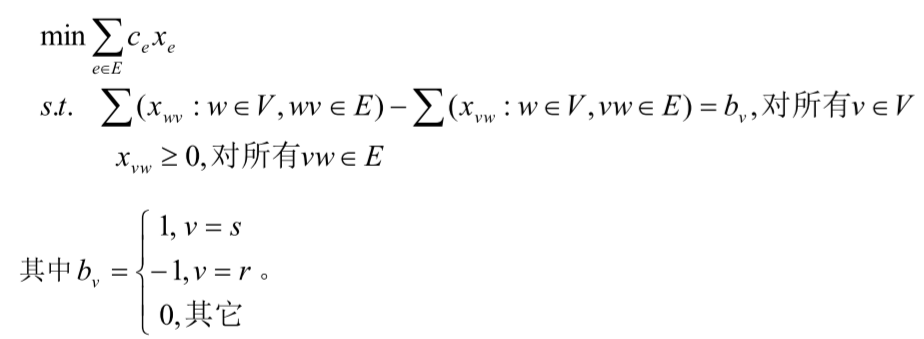其中： c e c_e e e 这条边的权值。 x e x_e 是代表这条边走不走。然后 x w v x_{wv} 就是指 e = ( w , v ) e=(w,v) 这条边走不走。
对于 b v b_v ，举个例子如下：以那个 b v = − 1 , v = r b_v=-1,v=r 为例，代入上面的约束条件，令 v = r v=r ,即源点，顶点1。则那个约束条件变为：

0 − x 12 − x 13 = − 1 0-x_{12}-x_{13}=-1

为什么前面那个求和是0？因为没有到源点的边，即没有入边。出边有两条，相减为-1，我想你已经想到了，这代表既然是求从源点1到4的最短路劲，那么这条路径要么经过2，要么经过3，即其中肯定要走而且只能是其中1个。

其他一次类推，比如终点表示必然要有而且仅有一条入边。中间节点表示从一条入边进，必然从一条出边出，且不能多，相抵了为0。

有人会问，这他喵不应该是整数规划嘛？怎么那个限制条件是 x v w ≥ 0 x_{vw}\ge 0 。的确整数规划0-1规划可以，但是在本文情况中，不必是，也可以得到最终的结果是取0或1，比较神奇。比如下面这样不会是最优解。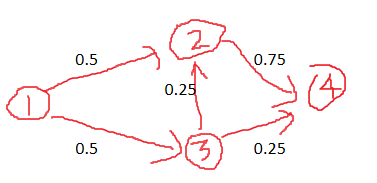虽然这个解 x v w x_{vw} 满足约束条件，但肯定不是最优解。别忘了我们还有一个最小化目标函数呢，你把所有边都走了遍，其中某些乘以了一个很大的权值，肯定不如最短路那个更小的权值。

展开全文组合最优化
• 数学模型-自己收藏的数学建模资料，用于数学建模，线性规划、单纯形法、最短路径、运输问题、整数规划、储存论、多目标规划数学建模
• 整数规划求解最短路径问题

千次阅读 2019-09-11 10:32:02
整数规划求解最短路径问题

介绍

最短路径就是从图中的某一对OD间找出一条权重之和最小的路径。常规的算法可以用Dijkstra算法、A*算法来求解，具体可看
去看最短路径算法实现

去看原文

模型有向图

在有向图中，并不是所有点对之间都有边连接，所以在建模的时候，我们需要将边不存在的点对之间的距离设置为无穷大，如下图，0号节点和3号节点之间不存在边，那么相应的距离矩阵d=无穷大，x = 0.代码

利用cplex求解代码

#java

IloCplex model = new IloCplex();

// define variables
IloIntVar[][] x = new IloIntVar[pNetwork.NodeSize()][pNetwork.NodeSize()]; //
for (int i = 0; i < x.length; i++) {
for (int j = 0; j < x.length; j++) {
x[i][j] = model.boolVar("X[" + i + ", " + j + "]"); // boolVar可以返回一个01的bool类型决策变量
}
}

// 出边和小于等于1
for (int i = 0; i < x.length; i++) {
IloLinearIntExpr r = model.linearIntExpr();
for (int j = 0; j < x.length; j++) {
}
}

// 入边和小于等于1
for (int j = 0; j < x.length; j++) {
IloLinearIntExpr r = model.linearIntExpr();
for (int i = 0; i < x.length; i++) {
}
}

// 除了起点和终点 出边和等于入边和
for (int m = 0; m < x.length; m++) {
if(m != pOriginNode.getIndex() && m != pDestNode.getIndex()){
IloLinearIntExpr r1 = model.linearIntExpr();
for (int j = 0; j < x.length; j++) {
}
IloLinearIntExpr r2 = model.linearIntExpr();
for (int j = 0; j < x.length; j++) {
}
}
}

// 起点的出边和为1
int m = pOriginNode.getIndex();
IloLinearIntExpr r1 = model.linearIntExpr();
for (int j = 0; j < x.length; j++) {
}
//起点的入边和为0
IloLinearIntExpr r2 = model.linearIntExpr();
for (int j = 0; j < x.length; j++) {
}

// 终点的出边和为0 入边和为1
m = pDestNode.getIndex();
r1 = model.linearIntExpr();
for (int j = 0; j < x.length; j++) {
}
//终点的入边和为1
r2 = model.linearIntExpr();
for (int j = 0; j < x.length; j++) {
}

// 不能经过节点本身
for (int i = 0; i < x.length; i++) {
IloLinearIntExpr r = model.linearIntExpr();
}

//边不存在的对应xij = 0
for (int i = 0; i < distanceMat.length; i++) {
for (int j = 0; j < distanceMat.length; j++) {
if(distanceMat[i][j] == Double.MAX_VALUE){
IloLinearIntExpr r = model.linearIntExpr();
}
}
}

// 最小化OD之间路径的权重之和
IloLinearNumExpr z = model.linearNumExpr();
for (int i = 0; i < x.length; i++) {
for (int j = 0; j < x.length; j++) {
if (i == j)
continue;
}
}

结果原文有源码，更多内容，请关注 地学数据处理分析。展开全文整数规划 最短路径 Cplex
• 优化方法的整数规划的分支定界方法 整数线性规划的求解 求解整数规划的割平面法 整数规划的分支定界法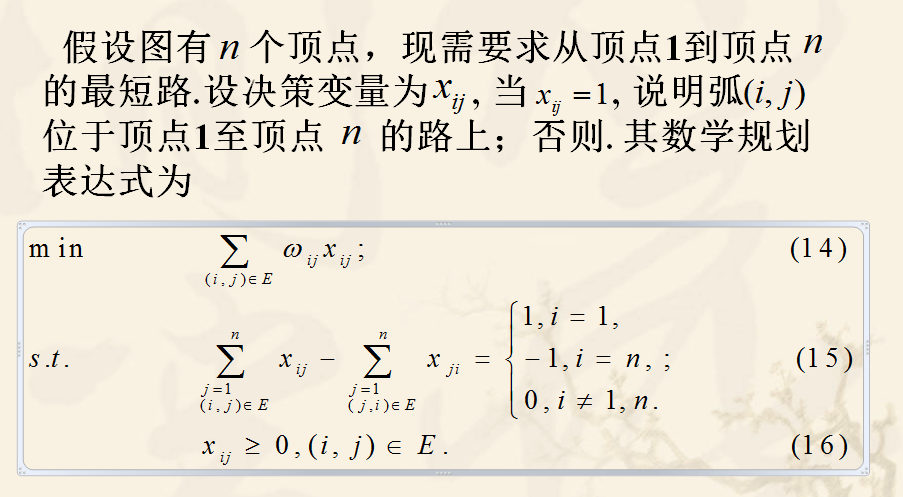约束条件的目的是：求得所有可能的从顶点1到顶点n的路径
其原理如下：
s.t.1
i=1时式子值为1：与点1相连的路径一定有一条为1，即点1一定可以走出去
i=n时式子值为-1：与点n相连的路径一定有一条为-1，即一定有路径通往点n（实际上此条件可省略，由于目标min）
i≠1，n时式子值为0,：路径可以通过约束1中创造的路径延续下去，直至点n

下面为一道最短路径的题目，将通过将上述数学表达式转化为lingo代码求解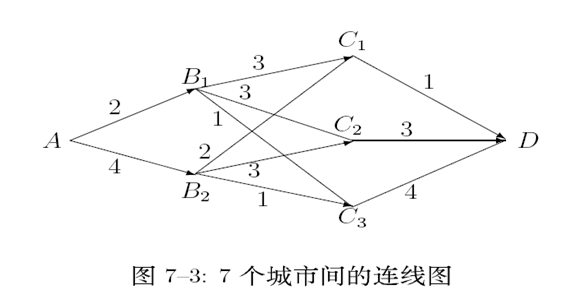model:
sets:
cities/A,B1,B2,C1,C2,C3,D/;!城市名称;
B2,C1 B2,C2 B2,C3 C1,D C2,D C3,D/:w,x;!w：两城市路径的权值，x：最短路径;
endsets

data:
w = 2  4  3  3  1  2  3  1  1  3  4;
enddata

n=@size(cities);

end

在上述程序中， n=@size(cities)是计
算集cities的个数，这里的计算结果是n=7, 这样编
写方法目的在于提高程序的通用性.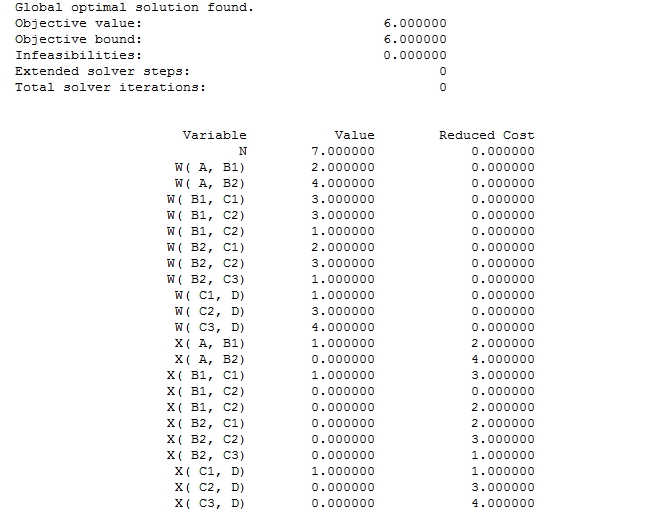运行结果，知道最短路径为6；
通过x(A,B1),x(B1,C1),x(C1,D)知道最短路径A——B1———C1———D

展开全文最短路径算法
• linprog只能求解目标函数为min的线性规划问题 linprog只能 直接 求解约束条件为⩽或=的问题 linprog的bounds参数如果不设定，所有变量将被视为自由变量 linprog函数的return是一个 scipy.optimize....

from pulp import *

my_LpProblem = LpProblem("test1", LpMaximize)

x = LpVariable("x", 0)

y = LpVariable("y", 0)

z = LpVariable("z", 0)

my_LpProblem += 2*x + 3*y + 1*z, "obj"

my_LpProblem += x+3*y+4*z >= 8, "c1"

my_LpProblem += 3*x+2*y+2*z >= 18, "c2"

my_LpProblem.solve()

# 打印出已经求解问题的状态

print("Status:", LpStatus[my_LpProblem.status])

# 打印出最优解

for v in my_LpProblem.variables():

print(v.name, "=", v.varValue)

# 打印最优目标函数值

print("objective=", value(my_LpProblem.objective))

from pulp import *

my_MipProblem = LpProblem("test1", LpMaximize)

solution = []

x = LpVariable("x", lowBound=0, cat=LpInteger)

y = LpVariable("y", cat=LpBinary)

z = LpVariable("z", lowBound=0)

my_MipProblem += 5 * x - 2 * y - 3 * z, "obj"

my_MipProblem += x + 2 * y + 3 * z == 9, "c1"

my_MipProblem += 2 * x + y + z == 5, "c2"

my_MipProblem.solve()

# 打印出已经求解问题的状态

print("Status:", LpStatus[my_MipProblem.status])

# 打印出最优解

for v in my_MipProblem.variables():

print(v.name, "=", v.varValue)

solution.append(v.varValue)

# 打印最优目标函数值

print("objective=", value(my_MipProblem.objective))

用scipy的linprog求解线性规划问题

1. 学习阅读官方文档
2. 注意
• linprog只能求解目标函数为min的线性规划问题
• linprog只能直接求解约束条件为⩽或=的问题
• linprog的bounds参数如果不设定，所有变量将被视为自由变量
• linprog函数的return是一个scipy.optimize.OptimizeResult(源代码)，它在本质上也是一个dict.

import numpy as np

from scipy.optimize import linprog

c = np.array([1, 2, 1])

A_ub = np.array([[-2, -3, -1]])

b_ub = np.array([-12])

A_eq = np.array([[1, 1, 3]])

b_eq = np.array()

my_linprog_result = linprog(c,A_ub,b_ub,A_eq,b_eq,bounds=((0,None),(0,None),(0,None)))

print(my_linprog_result)

my_solution = my_linprog_result.x

my_optimum_value = -my_linprog_result.fun

用scipy的linear_sum_assignment求解线性指派问题

1. 学习阅读scipy.optimize.linear_sum_assignment的官方文档
2. 注意
• linear_sum_assignment只能求解目标函数为最小值的线性指派问题
• 可以直接求解任务数与人数不对等的指派问题
• 输入参数必须为一个2D的numpy.array实例
• 返回的结果为最优指派对应在此2D array上的index

import numpy as np

from scipy.optimize import linear_sum_assignment

cost = np.array([[11, 10, 11, 3, 11], [8, 11, 10, 12, 14], [15, 5, 16, 2, 3], [15, 3, 5, 17, 14]])

time_array_standardized = np.array([[11, 10, 11, 3, 11], [8, 11, 10, 12, 14], [15, 5, 16, 2, 3], [15, 3, 5, 17, 14], [15, 3, 5, 17, 14]])

row_ind, col_ind = linear_sum_assignment(time_array_standardized)

minimum_time = time_array_standardized[row_ind, col_ind].sum()

print(row_ind)  # 开销矩阵对应的行索引

print(col_ind)  # 对应行索引的最优指派的列索引

print(time_array_standardized[row_ind, col_ind])  # 提取每个行索引的最优指派列索引所在的元素，形成数组

print(minimum_time)  # 数组求和

import numpy as np

from scipy.optimize import linear_sum_assignment

cost = np.array(

[[500, 550, 400, 480, 380], [590, 580, 480, 450, 470], [570, 420, 400, 410, 310], [590, 340, 580, 460, 380],

[530, 570, 490, 410, 520]])

cost1 = cost*-1

row_ind, col_ind = linear_sum_assignment(cost1)

maximum_sales = cost[row_ind, col_ind].sum()

print(row_ind)  # 开销矩阵对应的行索引

print(col_ind)  # 对应行索引的最优指派的列索引

print(cost[row_ind, col_ind])  # 提取每个行索引的最优指派列索引所在的元素，形成数组

print(maximum_sales)  # 数组求和

3.用networkx求解图论问题(1)

Python的Networkx包

NetworkX is a Python language software package for the creation, manipulation, and study of the structure, dynamics, and function of complex networks.

With NetworkX you can load and store networks in standard and nonstandard data formats, generate many types of random and classic networks, analyze network structure, build network models, design new network algorithms, draw networks, and much more.

预习内容：

2. 用Networkx的minimum_spanning_treeminimum_spanning_edges方法求解最小支撑树问题
3. 用Networkx的最短路求解方法求解最短路问题

4.   用Networkx的Maximum Flow算法方法求解网络最大流问题，要求：

• 能找到最小割

import networkx as nx

G = nx.DiGraph()

all_shortest_paths = []

all_shortest_paths = list(nx.all_shortest_paths(G, source=0, target=7, weight='weight', method='bellman-ford'))

shortest_path_length = nx.shortest_path_length(G, source=0, target=7, weight='weight', method='bellman-ford')

最大流问题

用Networkx的Maximum Flow算法方法求解以下网络最大流问题，要求：

节点的编号从0开始

返回G，其为下图所对应的DiGraph，其中弧上的权重用capacity表示.

返回max_flow_value，为最大流的流量(数值).

返回cut_set，为最小割集，可以是一个list或set.

求解下图中从v1

至v11的最大流及最小割，并思考最小割集是什么。图中弧上的数字表示其容量.

import networkx as nx

#构建graph

edges = [

[1, 4, 155],

[1, 3, 160],

[1, 2, 105],

[2, 5, 150],

[2, 6, 105],

[3, 5, 35],

[3, 6, 90],

[3, 7, 140],

[4, 6, 85],

[4, 7, 80],

[5, 8, 155],

[5, 9, 140],

[6, 8, 175],

[6, 9, 130],

[6, 10, 165],

[7, 9, 50],

[7, 10, 40],

[8, 11, 150],

[9, 11, 140],

[10, 11, 180]

]

G = nx.DiGraph()

for [start, end, capacity] in edges:

#求最大流

max_flow_value, flow_dict = nx.maximum_flow(G, 0, 10, capacity='capacity')

print("最大流值: ", max_flow_value)

print("最大流流经途径: ",flow_dict)

cut_value, partition = nx.minimum_cut(G, 0, 10, capacity='capacity')

reachable, non_reachable = partition

cut_set = set()

for u, nbrs in ((n, G[n]) for n in reachable):

cut_set.update((u, v) for v in nbrs if v in non_reachable)

print(sorted(cut_set))

cut_value == sum(G.edges[u, v]['capacity'] for (u, v) in cut_set)

展开全文• 最短路问题

2015-04-14 21:20:21
数学建模中最短路问题的原理及方法，使用MATLAB进行编程最短路问题
• % *lpint (BranchBound)- 线性整数规划 % *L01p_e - 0-1整数规划枚举法 % *L01p_ie - 0-1整数规划隐枚举法 % *bnb18 - 非线性整数规划(在MATLAB5.3使用) % *bnbgui - 非线性整数规划图形工具(在MATLAB5.3使用) % *...MATLAB
• Thorup发现了第一个确定性算法，用于解决线性时间和空间中具有正整数权重的无向图的经典单源最短路径问题。 该算法需要一个层次化的存储桶结构来标识必须访问顶点的顺序，而又不会破坏该时间范围，从而避免了...
• 线性规划与网络流24题 12】软件补丁 Description T 公司发现其研制的一个软件中有n 个错误，随即为该软件发放了一批共m 个补丁程序。每一个补丁程序都有其特定的适用环境，某个补丁只有在软件中包含某些错误而...
• 最短路，最大流，最大割；以及动态规划；网络评审技术等等。 可以说几乎囊括了运筹学的整个分支，其实每个章节拿出来都是可以大书特书的。 整数规划是其中的一个小章节，同时也是一类生活中非常常见的问题。生活中的...人工智能
• 我参加了 2019 年的数学建模比赛，所以... 优化问题：其中有线性规划、非线性规划整数规划、多目标规划、遗传算法；2.图论问题：其中有最短路问题、最小生成树、最大流、TSP 问题解法；3.数据分析：有拟合、插值、...
• lingo算法实现单源单宿最短路问题代码 线性规划的学习内容 有完整代码，包括带整数约束的
• 最短路径问题 什么是最短路径问题 最短路径问题和线性规划间的关系 Python调用Gurobi求解最短路径问题 Dijkstra’s Algorithm Dijkstra’s Algorithm 证明 Dijkstra’s Algorithm 实现python 算法
• 整数规划---0-1型整数规划

千次阅读 2018-12-08 19:46:00
2019独角兽企业重金招聘Python工程师标准>>> 0 - 1 型整数规划的解法   转载于:https://my.oschina.net/liyangke/blog/2984832
• [数模笔记]图论-最短路问题

千次阅读 2019-08-12 17:27:32
一、最短路问题概述 最短路问题即是寻找同一个网络中的两个节点之间的一条通路，使“消耗”在这条通路上的权重最小的问题，这里的权重可替换为最小距离、最短时间、最小成本等。常见的最短路问题可以体现为如下形式...最短路问题 Dijkstra算法 单源最短路问题 Floyd算法
• 线性规划线性规划（Linear Programming简称LP）是运筹学的一个重要分支，也是运筹学中理论成熟，应用广泛的方法之一。自1947年丹捷格提出一般线性规划问题的求解方法－－单纯形法之后，线性规划已被广泛地应用...
• 整数规划求解有向图最短路径问题环路解决方法 在有向图中，经常遇到给定起点和终点以及必经点，选择一条权重最小的路径这样的问题。这种问题可以看做是旅行商问题（tsp）的变种，tsp问题是一种组合爆炸问题，当规模...数学
• 假期 2020.01 .24 题目描述 ...在网络布线的工程中，有许多电缆，而电缆的粗细不同，流量与费用..."最短路数组:" endl ; cout " expense_choice [ ] = " ; for ( i = 1 ; i vertex_count ; ...网络 算法 数据结构
• 用Python求解线性规划问题

千次阅读 2020-03-15 10:31:00
线性规划简介及数学模型表示线性规划简介一个典型的线性规划问题线性规划模型的三要素线性规划模型的数学表示图解法和单纯形法图解法单纯形法使用python求解...
• 使用 短路&&,扩展赋值运算符,位运算 4、条件运算符(三目运算) 单目(一元)运算符 ：++,--,! 双目(二元)运算符 ：+，-，*，/，%，>,<, ... ... 三目(三元)运算符 ：?: 1、语法 条件?表达式1:表达式2; 条件...javascript
• 线性规划求解路径问题

千次阅读 2016-05-31 21:22:39
问题描述 给定一个带权重的有向图G=(V,E)，V为顶点集，E为有向边集，每一条有向边均有一个权重...整数规划求解思路记边的变量为ei e_i 对应权重为ci c_i , 点记为viv_i, 其出边记为vojivo_i^j， 其入边记为vijivi_i^j,
• 这一节课讲解了线性规划中的原始对偶方法（primal-dual method），并以最短路问题为例说明该方法的应用。 原始对偶方法 原始对偶方法利用的就是上一节课中讲到的互补松弛定理。我们首先找到对偶问题的一个可行解...
• Floyd算法其实是用到了动态规划的方法去解决图论问题，对于确定的起点与终点，我们可以通过状态的转移由之前求得的已知最短路来求得未知的最短路。如果要搞懂Floyd算法，你需要对于动态规划的知识点有所了解，否则...算法 动态规划 c++
• 一、问题描述在开始介绍最短路问题之前我们先来简单讨论网络流问题(network flow problems)在我们日常生活中，网络无处不在：为我们提供电力能源的电力网络，为我们提供方便通讯的电话网络，满足我们各种出行需求的...最短路算法的证明
• 参考文献：全国青少年信息学竞赛培训教材——复赛（陈合力 游光辉 编著） 一、概念在多阶段决策... 我们称这种解决多阶段决策优化的过程称为动态规划方法。 例如在一个m*n的迷宫中，从左下角走到右上角 可以看动态规划 线性动态规划
• 这一节课开始了整数...先从简单的线性整数规划开始。线性整数规划其实就是线性规划加上解必须为整数的限制，其基本形式为 \begin{matrix} \max\limits_x & c^Tx \\ \text{s.t.} & Ax \le b \\ & ...
• 前端面试题

万次阅读 多人点赞 2019-08-08 11:49:01
前端面试题汇总 ... 你做的页面在哪些流览器测试过？这些浏览器的内核分别是什么? 21 ... 21 Quirks模式是什么？它和Standards模式有什么区别 21 div+css的布局较table布局有什么优点？...img的alt与title有何异同？...前端面试题
• 第2章 整数线性规划 2．1 割平面法 2．2 分枝定界法 2．3 习题 第3章 无约束优化 3．1 数学预备知识 3．2 无约束优化问题的解 3. 3 用MATLAB优化工具箱解无约束优化 3．4 习题 第4章 非线性...matlab
• 整数规划的分支定界法；用MATLAB优化工具箱解线性规划；用Lingo软件求解；运输问题的数学模型；生产计划安排问题；分段函数的处理方法；人力资源安排问题；投资问题；最短路问题；设备更新问题...